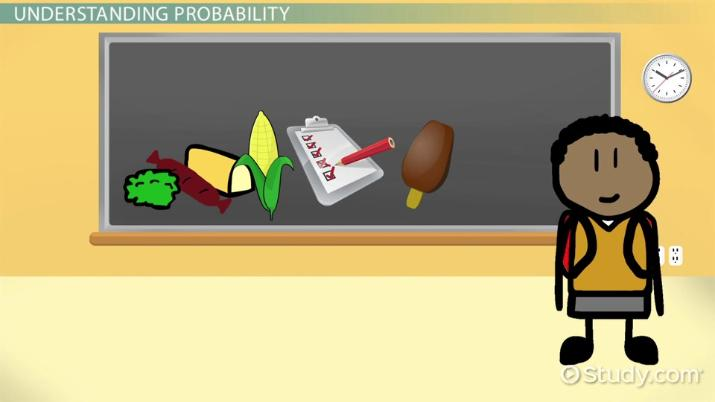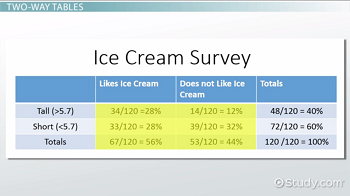# Using Two-Way Tables to Evaluate Independence

Lesson Transcript
Instructor: Cathryn Jackson

Cat has taught a variety of subjects, including communications, mathematics, and technology. Cat has a master's degree in education and is currently working on her Ph.D.

In statistics, two-way tables provide a method to visualize the frequencies of two categorical variables in a data set. Learn about using two-way tables to evaluate independence in variables. Understand probability, including independent and dependent probability, and recognize how to use two-way tables to predict probability. Updated: 10/11/2021

## Understanding Probability

Miquel is conducting research for his nutrition class. He is distributing a survey to all of the students in his school to determine if ice cream can make you taller. He collects all of the data and puts it into a table like this:

Likes Ice Cream Does Not Like Ice Cream Totals
Tall (>5.7) 34 14 48
Short (<5.7) 33 39 72
Totals 67 53 120

In this table, you can see all of the different types of data Miquel collected and calculate probabilities based off of this information. A probability is the likelihood or possibility of an outcome. For example, what is the likelihood that a randomly selected person over 5 ft. 7 in. in Miquel's school enjoys eating ice cream? Use the table to find your answer.

You can take the total number of students at Miquel's school and create a fraction with the number of people over 5 ft. 7 in. that enjoy eating ice cream. When you convert that into a percentage, you get your probability. For this example, we take 34, which is the number of people over 5 ft. 7 in. that enjoy eating ice cream, and divide by 120, which is the total number of students at Miquel's school. 34 / 120 = 28%. Therefore, you are 28% likely to meet a tall person that enjoys ice cream at Miquel's school.

So, the question is, does enjoying ice cream make you taller? Or, if you are taller, does that make you enjoy ice cream?

To answer these questions, you will need to understand independence and conditional probability. In this lesson, you will learn how to use a 2-way table to determine if a probability is independent. First, let's look at independent and dependent probability.An error occurred trying to load this video.

Try refreshing the page, or contact customer support.

Coming up next: Applying Conditional Probability & Independence to Real Life Situations

### You're on a roll. Keep up the good work!

Replay
Your next lesson will play in 10 seconds
• 0:25 Definition of Probability
• 1:39 Independent &…
• 2:28 Two-Way Tables
• 4:55 Evaluating Independence
• 7:06 Lesson Summary
Save Save

Want to watch this again later?

Timeline
Autoplay
Autoplay
Speed Speed

## Independent & Dependent Probability

When determining probability, you need to understand if the data you are collecting is being influenced by another event. There are two types of probability: independent and dependent.

A dependent event is when one event influences the outcome of another event in a probability scenario. For example, if you had a deck of cards and drew one card out of the deck, then this event would affect the probability of the next draw because you have fewer cards in your hand.

An independent probability is when the probability of an event is not affected by a previous event. For example, if a student were to flip a coin once and get heads, then flip the coin again, his first flip would not affect the outcome of the second flip. The chances of getting heads or tails are always the same: 50/50.

## Two-Way TablesThis is a 2-way table, which is just a table listing two categorical variables whose values have been paired. Each set of numbers in a 2-way table has a specific name. The middle cells are the joint frequency numbers. When analyzing data in a 2-way frequency table, you will be looking for joint relative frequency, which is the ratio of the frequency in a particular category to the total number of data values.

This is called joint frequency because you are joining one variable from the row and one variable from the column. In this example, the row variables are the heights and the column variables are whether or not the individual enjoys ice cream. Therefore, the number of tall people that enjoy ice cream would be considered a joint frequency.

The numbers in the column on the very right and the row on the very bottom are the marginal frequency numbers. When analyzing data in a 2-way frequency table, you will be looking for marginal relative frequency, which is the ratio of the sum of the joint relative frequency in a row or column to the total number of data values. When you think of marginal frequency, think of the margins on an English paper. The margins are the areas on the edges of the paper, and the marginal frequency numbers are the numbers on the edges of a table.

Conditional relative frequency numbers are the ratio of a joint relative frequency to related marginal relative frequency. For example, let's say you wanted to find the percentage of people that enjoy ice cream, given those people are short, which is a mathematical way of saying that you just want to find the percentage of short people who enjoy ice cream. You would then find the number of short people that selected that they enjoy ice cream and divide by the total number of short people in the survey. This is a similar set up to conditional probability, where the limitation, or condition, is preceded by the word given.

A conditional probability is the probability of a second event given a first event has already occurred. This means that one of the variables is dependent. For example, you could say that you have to be tall first before you can enjoy ice cream.

To unlock this lesson you must be a Study.com Member.

### Register to view this lesson

Are you a student or a teacher?